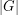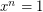order, dividing

Subgroup formed by elements of order dividing n ★★

Author(s): Frobenius

Conjecture

Supposeis a finite group, andis a positive integer dividing. Suppose thathas exactlysolutions to. Does it follow that these solutions form a subgroup of?

Keywords: order, dividing